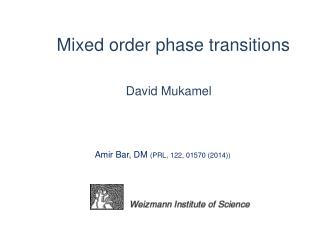# Mixed order phase transitions - PowerPoint PPT PresentationDownload PresentationMixed order phase transitions

Mixed order phase transitions
Download Presentation## Mixed order phase transitions

- - - - - - - - - - - - - - - - - - - - - - - - - - - E N D - - - - - - - - - - - - - - - - - - - - - - - - - - -
##### Presentation Transcript

1. Mixed order phase transitions David Mukamel Amir Bar, DM (PRL, 122, 01570 (2014))

2. Phase transitions of mixed order (a) diverging length as in second order transitions or (b) discontinuous order parameter as in first order transitions

3. Examples: 1d Ising model with long range interactions non-soluble but many of its properties are known 2. Poland-Scheraga (PS) model of DNA denaturation 3. Jamming transition in kinetically constrained models • Toninelli, Biroli, Fisher (2006) 4. “Extraordinary transition” in network rewiring Liu, Schmittmann, Zia (2012)

4. IDSI : Inverse Distance Square Ising model For the model has an ordering transition at finite T A simple argument: ++++++++++++-----------------++++++++++++++++++++ 1 L Anderson et al (1969, 1971); Dyson (1969, 1971); Thouless (1969); Aizenman et al (1988)…

5. model is special The magnetization m is discontinuous at (“Thouless effect”) Thouless (1969), Aizenman et al (1988) KT type transition, Cardy (1981) Phase diagram H T IDSI Fisher, Berker (1982)

6. Dyson hierarchical version of the model (1971) Mean field interaction within each block The Dyson model is exactly soluble demonstrating the Thouless effect

7. Exactly soluble modification of the IDSI model microscopic configuration: +++++++++------------------------+++++++++++++++++--------------------- The interaction is in fact not binary but rather many body.

8. Summery of the results diverging correlation length at with nonuniversal Extreme Thouless effect with Phase diagram H T The model is closely related to the PS model of DNA denaturation

9. The energy of a domain of length Interacting charges representation: Charges of alternating sign (attractive) on a line Attractive long-range nearest-neighbor interaction Chemical potential --suitable representation for RG analysis --similar to the PS model

10. Analysis of the model Grand partition sum Polylog function

11. Polylog function is the closest pole to the origin ferromagnetic coupling

12. Phase transition: Unlike the PS model the parameter c is not universal

13. Nature of the transition Domain length distribution Close to : characteristic length

14. Two order parameters number of domains magnetization 1. order parameter Where at for for is finite is continuous is discontinuous in both cases

15. 2. order parameter is the magnetic field symmetry) either or Extreme Thouless effect

16. Phase diagram I n is continuous II and III n is discontinuous

17. phase diagram

18. Canonical analysis Free energy

20. c=2.5

21. Finite L correction:

22. c=2.5

23. Finite L corrections c=2.5 L=1000

24. Renormalization group - charges representation + - + - y - fugacity a - short distance cutoff Length rescaling This can be compensated by y rescaling

25. + - + - The integral scales like hence it does not renormalize c . Rather it renormalizes y.

26. Renormalization group equations compared with the Kosterlitz-Thouless model:

27. In the KT case: + - + - Contribution of the dipole to the renormalized partition sum: (Cardy 1981) renormalizes c.

28. Line of fixed points

29. Coarsening dynamics • Particles with n-n logarithmic interactions • Biased diffusion, annihilation and pair creation

30. Coarsening dynamics The coarsening is controlled by the T=0 (y=0) fixed point + Like the dynamics of the T=0 Ising model

31. Coarsening dynamics Expected scaling form - number of domains with

32. L=5000 c=1.5 z=2 z=1.5

33. with - Voter model (y=0, fixed c)

34. Summary Some models exhibiting mixed order transitions are discussed. A variant of the inverse distance square Ising model is studied and shown to have an extreme Thouless effect, even in the presence of a magnetic field Relation to the IDSI model is studies by comparing the renormalization group transformation of the two models. The model exhibits interesting coarsening dynamics at criticality.

35. Domain representation of the Ising model (Fortuin-Kasteleyn representation) defines a graph on the vertices The sum is over all graphs E

36. A graph can be represented as composed of sub-graphs separated by “breaking points” One has to calculate - the probability that the distance between adjacent breaking points is